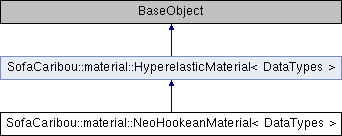Caribou
SofaCaribou::material::NeoHookeanMaterial< DataTypes > Class Template Reference
Inheritance diagram for SofaCaribou::material::NeoHookeanMaterial< DataTypes >:## Public Member Functions

SOFA_CLASS (SOFA_TEMPLATE(NeoHookeanMaterial, DataTypes), SOFA_TEMPLATE(HyperelasticMaterial, DataTypes))

void before_update () override
This is called just before the material is updated on every points (usually just before a Newton step). More...

Real strain_energy_density (const Real &J, const Eigen::Matrix< Real, Dimension, Dimension > &C) const override
Get the strain energy density Psi from the right Cauchy-Green strain tensor C. More...

virtual Eigen::Matrix< Real, Dimension, Dimension > PK2_stress (const Real &J, const Eigen::Matrix< Real, Dimension, Dimension > &C) const override
Get the second Piola-Kirchhoff stress tensor from the right Cauchy-Green strain tensor C.

virtual Eigen::Matrix< Real, 6, 6 > PK2_stress_jacobian (const Real &J, const Eigen::Matrix< Real, Dimension, Dimension > &C) const override
Get the jacobian of the second Piola-Kirchhoff stress tensor w.r.t the right Cauchy-Green strain tensor C.Public Member Functions inherited from SofaCaribou::material::HyperelasticMaterial< DataTypes >
SOFA_CLASS (SOFA_TEMPLATE(HyperelasticMaterial, DataTypes), sofa::core::objectmodel::BaseObject)

## Additional Inherited MembersStatic Public Member Functions inherited from SofaCaribou::material::HyperelasticMaterial< DataTypes >
static std::string templateName (const HyperelasticMaterial< DataTypes > *=nullptr)
Return the data type (ex. More...

static bool canCreate (HyperelasticMaterial< DataTypes > *o, sofa::core::objectmodel::BaseContext *context, sofa::core::objectmodel::BaseObjectDescription *arg)
Check if we can create a HyperelasticMaterial<DataTypes> with the given template argument (from the scene parser). More...

## ◆ before_update()

template<class DataTypes >
 void SofaCaribou::material::NeoHookeanMaterial< DataTypes >::before_update ( )
inlineoverridevirtual

This is called just before the material is updated on every points (usually just before a Newton step).

It can be used to update some coefficients that will be used on material points (for example, compute the parameters mu and lambda from the young modulus and poisson ratio given as data arguments).

Reimplemented from SofaCaribou::material::HyperelasticMaterial< DataTypes >.

## ◆ strain_energy_density()

template<class DataTypes >
 Real SofaCaribou::material::NeoHookeanMaterial< DataTypes >::strain_energy_density ( const Real & J, const Eigen::Matrix< Real, Dimension, Dimension > & C ) const
inlineoverridevirtual

Get the strain energy density Psi from the right Cauchy-Green strain tensor C.

Psi(C) = mu/2 (tr(C)-3) - mu ln(J) + lambda/2 (ln(J))^2

The documentation for this class was generated from the following file: